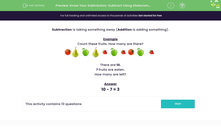Know Your Subtraction: Subtract Using Statements (2)

In this worksheet, students use statements to solve questions on subtraction.Key stage:  KS 1

Curriculum topic:   Number: Addition and Subtraction

Curriculum subtopic:   Make Mathematical Statements Using +, - and =

Difficulty level:Worksheet Overview

Example

Count these fruits. How many are there?There are 10.

7 fruits are eaten.

How many are left?